# Diffusion process

2010 Mathematics Subject Classification: Primary: 60J60 [MSN][ZBL]

A continuous Markov process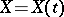with transition density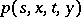which satisfies the following condition: There exist functions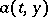and, known as the drift coefficient and the diffusion coefficient respectively, such that for any,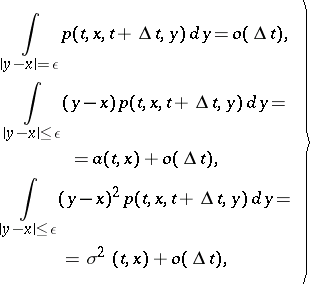(1)

it being usually assumed that these limit relations are uniform with respect toin each finite intervaland with respect to,. An important representative of this class of processes is the process of Brownian motion, which was originally considered as a mathematical model of diffusion processes (hence the name "diffusion process" ).

If the transition densityis continuous inandtogether with its derivatives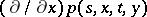and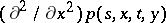, it is the fundamental solution of the differential equation(2)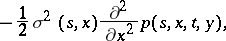which is known as the backward Kolmogorov equation (cf. also Kolmogorov equation).

In the homogeneous case, when the drift coefficient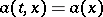and the diffusion coefficientare independent of the time, the backward Kolmogorov equation for the respective transition density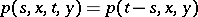has the form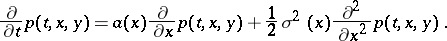If the transition densityhas a continuous derivative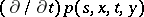inandsuch that the functionsand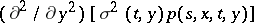are continuous in, it is the fundamental solution of the differential equation(3)known as the Fokker–Planck equation, or the forward Kolmogorov equation. The differential equations (2) and (3) for the probability density are the fundamental analytic objects of study of diffusion processes. There is also another, purely "probabilistic" , approach to diffusion processes, based on the representation of the process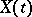as the solution of the Itô stochastic differential equation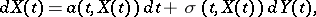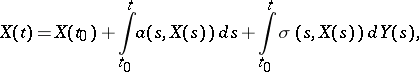where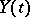is the standard process of Brownian motion. Roughly speaking,is considered to be connected with some Brownian motion processin such a way that if, then the incrementduring the next period of time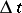is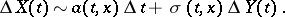If this asymptotic relation is understood in the sense that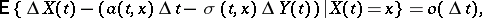whereare magnitudes of the same type as in equations (1), theunder consideration will constitute a diffusion process in the sense of this definition as well.

Multi-dimensional diffusion process is the name usually given to a continuous Markov processin an-dimensional vector space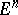whose transition density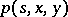satisfies the following conditions: For any,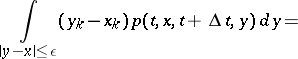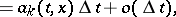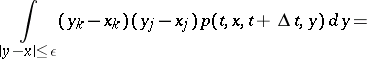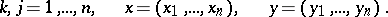The vectorcharacterizes the local drift of the process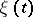, and the matrix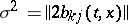,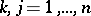, characterizes the mean square deviation of the random processfrom the initial positionin a small period of time betweenand.

Subject to certain additional restrictions, the transition densityof a multi-dimensional diffusion process satisfies the forward and backward Kolmogorov differential equations: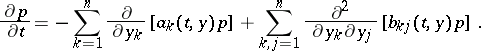A multi-dimensional diffusion processmay also be described with the aid of Itô's stochastic differential equations: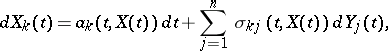where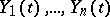are mutually-independent Brownian motion processes, whileare the eigen vectors of the matrix.

How to Cite This Entry:
Diffusion process. Encyclopedia of Mathematics. URL: http://encyclopediaofmath.org/index.php?title=Diffusion_process&oldid=26405
This article was adapted from an original article by Yu.A. Rozanov (originator), which appeared in Encyclopedia of Mathematics - ISBN 1402006098. See original article